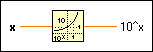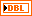# Power Of 10 Function

## LabVIEW 2018 Help

Edition Date: March 2018
Part Number: 371361R-01
View Product InfoLabVIEW 2016 HelpLabVIEW 2017 HelpLabVIEW 2018 HelpLabVIEW 2019 HelpLabVIEW 2020 Help

Owning Palette: Exponential Functions

Requires: Base Development System

Computes 10 raised to the x power.

The connector pane displays the default data types for this polymorphic function.

Examplex can be a scalar number, array or cluster of numbers, array of clusters of numbers, and so on.10^x is of the same numeric representation as x. When x is of the form x = a + bi, that is, when x is complex, the following equation defines 10^x:10^x = 10^a * 10^(bi) = 10^a * (cos(b * ln(10)) + i sin (b * ln(10)))

## Example

Refer to the Exponential VI in the labview\examples\Mathematics\Elementary & Special Functions\Exponential Functions directory for an example of using the Power Of 10 function.